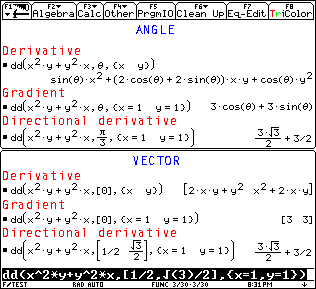## Texas Instruments TI-89 and TI-92 Plus Computer

Last page update: Sunday, November 21, 1999

 Directional Derivative and Gradient - for the TI-89 and TI-92 Plus Version 0.1   (Thursday, November 11, 1999) Download [ dd.89f  | dd.9xf ] (1,421 bytes) Description Directional derivative or gradient from either an angle (2-var) or vector (all). Note: This function is still being developed and may have errors. Screen shotsThis is an altered image. Documentation Usage:  dd(Expr,AngleOrVector,VarPointList)  Expr: A continuous function.  AngleOrVector: A vector matrix or an angle. A vector is a one dimensional matrix, either row ([x,y]) or column ([x;y]). It can be the zero vector (, [0;0], [0,0,0], etc.). The angle can be in either degrees or radians if the appropriate symbol is used ( ° , r ), or in the default unit if no symbol is used, or it can be an undefined variable.  VarPointList: Must list all variables, {x, y,...}, and may indicate the point of evaluation, {x=Px, y=Py,...}.  Return value:  The derivative: If AngleOrVector is a zero vector, , or evaluates to a variable, and VarPointList does not include the point. If AngleOrVector is a vector, the derivative will be returned as a vector.  The gradient: If AngleOrVector is a zero vector, , or evaluates to a variable, and VarPointList includes the point. If AngleOrVector is a vector, the gradient will be returned as a vector.  The directional derivative: If AngleOrVector is an angle or a non-zero vector.  Examples:  ANGLE  1. dd(x2y+xy2,u,{x,y)) Derivative       x2 sin(u)+2xy(cos(u)+sin(u))+cos(u)y2  2. dd(x2y+xy2,u,{x=1,y=1)) Gradient       3 cos(u)+3 sin(u)  3. dd(x2y+xy2,pi/3,{x=1,y=1)) Directional derivative       3*sqrt(3)/2+3/2  VECTOR   1. dd(x2y+xy2,,{x,y)) Derivative       [2xy+y2 , x2+2xy]  2. dd(x2y+xy2,,{x=1,y=1)) Gradient       [3 , 3]  3. dd(x2y+xy2,[1/2 , sqrt(3)/2],{x=1,y=1)) Directional derivative       3*sqrt(3)/2+3/2  4. dd(x2y+xy2-z,[1, 1, 0],{x=1,y=1,z=0)) Directional derivative       3*sqrt(2)